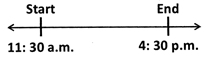Courses

# Test: Time - 2

## 10 Questions MCQ Test Mathematics for Class 4: NCERT | Test: Time - 2

Description
This mock test of Test: Time - 2 for Class 4 helps you for every Class 4 entrance exam. This contains 10 Multiple Choice Questions for Class 4 Test: Time - 2 (mcq) to study with solutions a complete question bank. The solved questions answers in this Test: Time - 2 quiz give you a good mix of easy questions and tough questions. Class 4 students definitely take this Test: Time - 2 exercise for a better result in the exam. You can find other Test: Time - 2 extra questions, long questions & short questions for Class 4 on EduRev as well by searching above.
QUESTION: 1

### The figure shows a time line.Find the duration between the two given times.

Solution:

From the given figure, start time = 11:30 a.m. End time = 4:30 p.m.
∴ The required duration = 4:30 p.m.−11:30 a.m.=5 hours

QUESTION: 2

### How is half past 9 in the morning written?

Solution:

Half past 9 in the morning means 30 minutes after 9 in the morning which is written as 9: 30 a.m.

QUESTION: 3

### How is 10 minutes past 8 in the evening written?

Solution:

10 minutes past 8 in the evening is written as 8:10 p.m.

QUESTION: 4

What time does 23:45 hours denote?

Solution:
QUESTION: 5

How is 10: 40 a.m. written in 24 hour clock time?

Solution:
QUESTION: 6

What fraction of 1 min 20 s is 45 s?

Solution:

The time span of 1 minute and 20 seconds contains 80 seconds in total. To determine the fraction we would divide 80 seconds by 45 seconds . 45 / 80 reduces to 9 / 16 . That is your answer ; 9/16 .

QUESTION: 7

What time is it 3 hours before 4:40 a.m.?

Solution:
QUESTION: 8

What time is it 10 minutes after 5:55 p.m.?

Solution:
QUESTION: 9

How many days are there in a year?

Solution:
QUESTION: 10

Which of the following numbers divides a leap year exactly?

Solution:

A leap year is exactly divisible by 4.